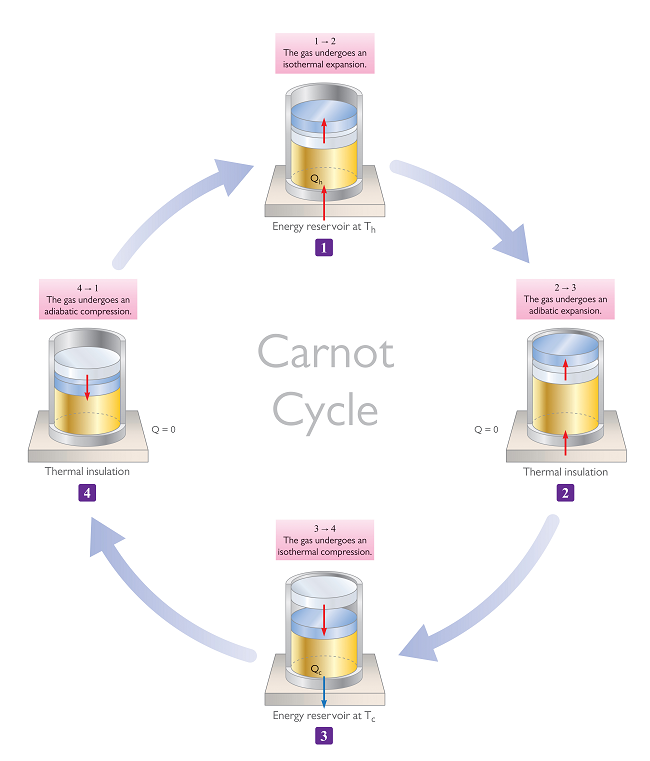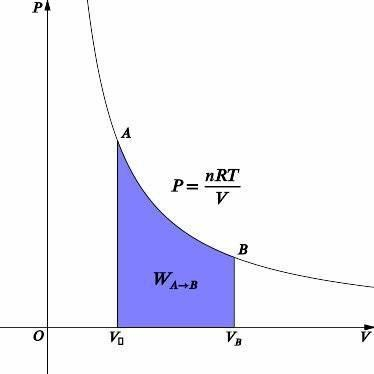# Difference between Isothermal and Adiabatic Processes

## Introduction

The division of the sciences we know about so far does not stop at Physics, Chemistry, and Biology. As we delve deeper into these realms, we come across an increasing need to classify our knowledge into various sub-branches under these three main headings of Physics, Chemistry, and Biology. For example, organic chemistry deals with carbon-based compounds and their properties.

One such branch of Physics is what we call thermodynamics. While the field of thermodynamics is incredibly vast, we will try to summarize its definition here and then discuss two main types of thermodynamic processes, namely, isothermal, and adiabatic.

## What is Thermodynamics?

The Encyclopedia Britannica defines Thermodynamics as the “science of the relationship between work, heat, energy, and temperature”. This is the most succinct description of what thermodynamics is about.

The word thermodynamics is itself a compound of therme and dynamis, which are Greek words meaning heat and power, respectively. Thus, etymologically speaking, thermodynamics is the study of the power that heat has.

In thermodynamics, we study how work, temperature, and heat are connected to energy and entropy. We also study how the physical properties of matter and radiation affect heat and temperature. The entirety of what we know about thermodynamics can be quickly summarized in a set of four rules, commonly known as the laws of thermodynamics.

## Laws of Thermodynamics

The laws of thermodynamics can be stated in various forms. However, they are all universally valid and exceptions to their implications have not been found. Interestingly, the first of these laws is called the Zeroth law, instead of being called “the first law”. Let us discuss in detail:

### Zeroth Law

The zeroth law states the equivalence of thermal equilibrium and provides an empirical way to define what temperature is. As per the Zeroth law, if you were to take two systems that were thermally stable with a third system, they would also have to be in thermal equilibrium with each other. This law is the same as the mathematical expression of equivalence. If A=C and B=C, then A=B.

### First Law

The first law expresses the relation between the internal energy of the system with heat and work. Its mathematical statement is as follows:

$\mathrm{\Delta U = Q-W}$

Here, ΔU is the change in internal energy of the system, Q is the heat energy gained, and W is the work done by the system on its surroundings.

### Second Law

The second law establishes the impossibility of 100% efficiency. It states that:

“In a cyclic process, all the heat energy cannot be converted into work.”

The second law can be used to predict whether a process is allowed or not. Sometimes, a certain process follows the conservation of energy but is still forbidden due to its invalidation of the second law.

### Third Law

The third law predicts the behavior of systems as they reach absolute zero. In simple terms, it says that when the temperature of a system approaches absolute zero, all processes cease, and its entropy starts to approach a constant value that may or may not be zero.

## What is Isothermal Process?

A process wherein, the temperature of the system does not change, is termed an isothermal process. The word isothermal is derived from the Greek roots “isos” and “therme”, meaning equal and heat, respectively.

Here’s the interesting thing, though. Although we are saying that the temperature must be constant, no limitations have been made on the heat flow in or out of the system.

## Examples of Isothermal Process

### Phase Change

One of the most common examples of an isothermal process is that of a change of phase. When a solid changes into a liquid, or a liquid into vapor, heat is gained by the system. However, melting and evaporation occur isothermally.

### Carnot Engines

A Carnot engine has various stages, and two of these stages involve an isothermal process. Refrigerators are based on the Carnot Cycle and thus, they also involve isothermal stages.## Expression for Work Done in Isothermal Process

The thermodynamic expression for work done is given below.

$\mathrm{W=\int\:P \cdot dV}$In an isothermal process, we know that the temperature is constant. The graph for an isothermal process is given above. Using the ideal gas equation, we can relate pressure and volume.

$\mathrm{PV = nRT}$

$\mathrm{P=\frac{nRT}{V}}$

Now, we can insert this value into the expression for work done.

$\mathrm{W=\int\:\frac{nRT}{V} dV}$

Since the number of moles, the temperature, and R is constants, we can leave them out of the integration. Thus,

$\mathrm{W=nRT\:ln \:ln V |_{V_1}^{V_2}=nRT\:ln\:ln (\frac{V_2}{V_1})}$

Therefore, the work done in an isothermal process is given by

$\mathrm{W=nRT\:ln\:ln(\frac{V_2}{V_1})}$

The term “adiabatic” arises out of the Greek term “adiabatos”, which means impassable. In this process, there is no transfer of heat or matter takes place. The system does not gain or lose heat energy from or to its surroundings, and neither does it exchange matter.

Processes that occur extremely rapidly gain occur with almost no heat exchange and thus, an “adiabatic approximation” can be made in various situations, making them easy to solve.

### Ice in an Icebox

To keep soft drinks cold in an icebox, we put ice inside it and close it. Now, heat does not flow in or out of the system and thus, the system becomes adiabatic.

### Propagation of Sound in Air

While flowing through a gas, the speed of sound is quite rapid and thus, there is no time for heat exchange to occur. Thus, the propagation of sound in a gas is an adiabatic process.

## Work Done in Adiabatic Process

For an adiabatic process, the relation between pressure and volume is as follows:

$\mathrm{PV^{\gamma}=K}$

Where K is a constant. We can use this relation to solve the expression for work done

$\mathrm{W=\int\:\:P \cdot dV=K \int \frac{1}{V-\gamma}dV=\frac{K}{1-\gamma}(V_2^{1-\gamma}-V_1^{1-\gamma})}$

The expression for K can be arrived at using the ideal gas equation

$\mathrm{P_1 V_1^{\gamma}=P_2 V_2^{\gamma}=K}$

$\mathrm{PV=nRT}$

Thus,

$\mathrm{W=\frac{1}{1-\gamma}(P_2 V_2-P_1 V_1)}$

## Conclusion

Thermodynamics is the study of heat, work, temperature, and their relation to energy, entropy, and matter. Our knowledge of thermodynamics can be summarized in a set of four rules known as the laws of thermodynamics.

An isothermal process occurs without a temperature change, even if heat is lost or gained by the system. A common example is the change of phase of solids into liquids via melting. The work of an isothermal process is given by the following equation:

$\mathrm{W=nRT\:ln\:ln(\frac{V_2}{V_1})}$

An adiabatic process is defined as one which occurs without conveying heat or matter between the system and its environment. The propagation of sound in air occurs too rapidly for heat exchange to occur and thus, it is adiabatic. The expression for work done in such a case is:

$\mathrm{W=\frac{1}{1-\gamma}(P_2 V_2-P_1 V_1)}$

## FAQs

Q1. What is the basic difference between an isothermal and an adiabatic process?

Ans. Isothermal processes occur at a constant temperature, but heat exchange can take place. However, no heat exchange must take place in an adiabatic process, even if a temperature change is involved.

Q2. How can heat exchange occur in an isothermal process?

Ans. When heat flows in or out of the system at an extremely slow pace, no temperature change occurs and thus, the process becomes isothermal.

Q3. Is a refrigerator an adiabatic system?

Ans. No. A refrigerator is an isothermal system. The contents of the refrigerator are maintained at a constant temperature and heat is pumped out.

Q4. What sign convention is followed for the work done?

Ans. In physics, the thermodynamic work done is positive if work is done by the system and is negative if work is done on the system.

Q5. What is the gamma $\mathrm{(\gamma)}$ term in the adiabatic process equation?

Ans. $\mathrm{\gamma}$ represents the adiabatic index. To calculate it, simply divide the specific heat at constant pressure by the specific heat at constant volume. That is, $\mathrm{\gamma=\frac{C_p}{C_v}}$